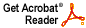# Subspaces of L^p(G) spanned by characters

Joel H. Shapiro

Israel J. Math 29 (1978), 248--264

Abstract: Let G be an infinite compact abelian group, mu a Borel measure on G with spectrum E, and 0 < p < 1. We show that if mu is not absolutely continuous with respect to Haar measure, then L^p_E(G), the closure in L^p(G) of the E-trigonometric polynomials, does not have enough continuous linear functionals to separate points. If mu is actually singular, then L^p_E(G) does not have any nontrivial continuous linear functionals at all. Our methods recover the classical F. and M. Riesz theorem, and a related several variable result of Bochner; they reveal the existence of small sets of characters that span L^p(T), where T is the unit circle; and they show that, for 0<p<1, the H^p spaces of the "big disc algebra" have one-dimensional dual.
Download .pdf file (556KB)